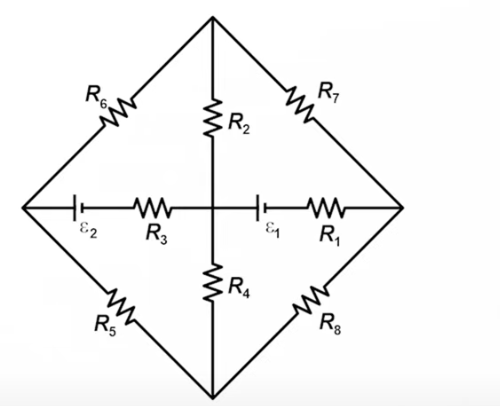# Symmetry in Circuits | Doubt regarding current flow

Doubt: Since the potential difference across the top point and bottom most point is zero, that is, the points are at same potential, there shouldn’t be any current flowing through the resistors R_2 and R_4. But since the potential difference across the resistor R_2 is not zero so current should flow through it. This is contradicting. Please explain.

Problem: All resistors are of 1 \Omega each and batteries E_1 and E_2 are of EMF 12V and 6V each. Find current through resistors R_!, R_3, R_5.I’ll be answering according to this picture

There is a nod B between nods A and C, so this rule does not apply here

Not exactly. If you add 5+3, then substract 3 from the previous result you get 5. Same thing happens here. Circuit is symmetrical across axis with EMFs, so \varphi_B - \varphi_A = \varphi_B - \varphi_C, meaning current floats from the nod B to the A and C, or vice-versa.

Side note. I kindly ask not to mention certain users after such a short time after posting the question. We won’t answer questions sooner than we will be able to anyway, and some of the active physicists are on a camp right now, so they cannot check the forum as much

6 лайков

Thanks!

I am sorry.

1 лайк

There is a nod B between nods A and C, so this rule does not apply here

Ah the potentials after calculating come out to be equal in the end. Thats where my main confusion lies… i know about the node voltage method. But here the case is different. I am able to get in mathematically but intuitively it is still not that clear

This might help (red arrows show the direction of the current). If we rotate the circuit 180 ^\circ relative to ED axis, it will stay the same (since all resistors are 1 \Omega), so we can conclude that the currents float symmetrically, too. Potential differences are found easily using Ohm’s or Kirchhoff’s laws.

1. currents across BC and AB might have the opposite direction, but it won’t affect the logic ↩︎

3 лайка

Please refrain from tagging people who you’d like to answer your question. If they’ll want to answer someone’s question, they’ll open a list of unread topics and see if there’s any topic name (yet another reason to keep it informative) they deem interesting, they are capable of opening the topic and reading it. Tagging someone does not increase the chance of seeing the topic nor the probability of answering it (and in fact may even lower it, as it may be considered annoying).

You shouldn’t expect to receive an answer to your topic immediately (or even within the same day)

5 лайков